# Difference between revisions of "06-240/Classnotes For Tuesday October 3"

Jump to: navigation, search

### Links to Classnotes

Definition.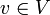$v\in V$is a linear combination of elements in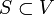$S\subset V$ if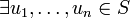$\exists u_1,\ldots,u_n\in S$ and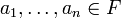$a_1,\dots,a_n \in F$ such that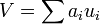$V=\sum a_i u_i$

Example. In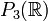$P_3(\mathbb{R})$,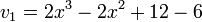$v_1=2x^3-2x^2+12-6$ is a linear combination of: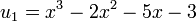$u_1=x^3-2x^2-5x-3$ and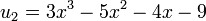$u_2=3x^3-5x^2-4x-9$ but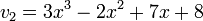$v_2=3x^3-2x^2+7x+8$ is not.

Why?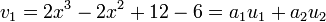$v_1=2x^3-2x^2+12-6=a_1u_1+a_2u_2$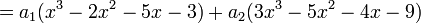$=a_1(x^3-2x^2-5x-3)+a_2(3x^3-5x^2-4x-9)$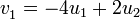$v_1^{}=-4u_1+2u_2$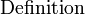$\mbox{Definition}{}_{}^{}$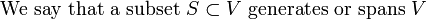$\mbox{We say that a subset }S\subset V\mbox{ generates or spans }V$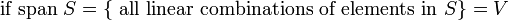$\mbox{ if span }S=\lbrace\mbox{ all linear combinations of elements in } S\rbrace=V{}_{}^{}$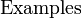$\mbox{Examples}{}_{}^{}$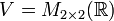$V=M_{2\times 2}(\mathbb{R})$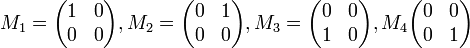$M_1=\begin{pmatrix}1&0\\0&0\end{pmatrix}, M_2=\begin{pmatrix}0&1\\0&0\end{pmatrix}, M_3=\begin{pmatrix}0&0\\1&0\end{pmatrix}, M_4\begin{pmatrix}0&0\\0&1\end{pmatrix}$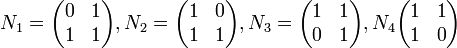$N_1=\begin{pmatrix}0&1\\1&1\end{pmatrix}, N_2=\begin{pmatrix}1&0\\1&1\end{pmatrix}, N_3=\begin{pmatrix}1&1\\0&1\end{pmatrix}, N_4\begin{pmatrix}1&1\\1&0\end{pmatrix}$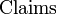$\mbox{Claims}{}_{}^{}$

1.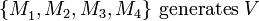$\lbrace M_1^{},M_2,M_3,M_4\rbrace\mbox{ generates }V$
2.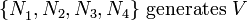$\lbrace N_1^{},N_2,N_3,N_4\rbrace\mbox{ generates }V$
3.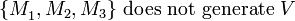$\lbrace M_1^{},M_2,M_3\rbrace\mbox{ does not generate }V$
4.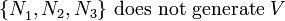$\lbrace N_1^{},N_2,N_3\rbrace\mbox{ does not generate }V$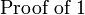$\mbox{Proof of 1}{}_{}^{}$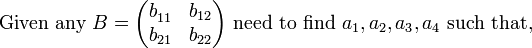$\mbox{Given any }B=\begin{pmatrix}b_{11}^{}&b_{12}\\b_{21}&b_{22}\end{pmatrix}\mbox{ need to find }a_1,a_2,a_3,a_4\mbox{ such that,}$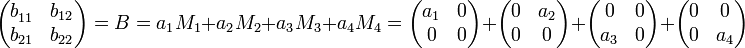$\begin{pmatrix}b_{11}^{}&b_{12}\\b_{21}&b_{22}\end{pmatrix}=B=a_1M_1+a_2M_2+a_3M_3+a_4M_4=\begin{pmatrix}a_1&0\\0&0\end{pmatrix} +\begin{pmatrix}0&a_2\\0&0\end{pmatrix} +\begin{pmatrix}0&0\\a_3&0\end{pmatrix} +\begin{pmatrix}0&0\\0&a_4\end{pmatrix}$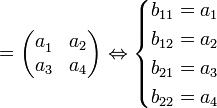$=\begin{pmatrix}a_1^{}&a_2\\a_3&a_4\end{pmatrix}\Leftrightarrow \begin{cases}b_{11}=a_1\\b_{12}=a_2\\b_{21}=a_3\\b_{22}=a_4\end{cases}$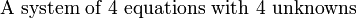$\mbox{A system of 4 equations with 4 unknowns}{}_{}^{}$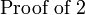$\mbox{Proof of 2}{}_{}^{}$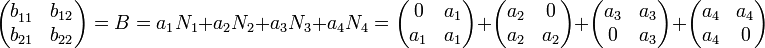$\begin{pmatrix}b_{11}^{}&b_{12}\\b_{21}&b_{22}\end{pmatrix} =B=a_1N_1+a_2N_2+a_3N_3+a_4N_4= \begin{pmatrix}0&a_1\\a_1&a_1\end{pmatrix} +\begin{pmatrix}a_2&0\\a_2&a_2\end{pmatrix} +\begin{pmatrix}a_3&a_3\\0&a_3\end{pmatrix} +\begin{pmatrix}a_4&a_4\\a_4&0\end{pmatrix}$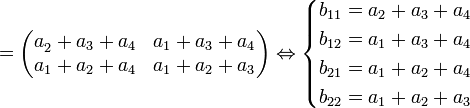$=\begin{pmatrix}a_2^{}+a_3+a_4&a_1+a_3+a_4\\a_1+a_2+a_4&a_1+a_2+a_3\end{pmatrix}\Leftrightarrow \begin{cases}b_{11}=a_2+a_3+a_4\\b_{12}=a_1+a_3+a_4\\b_{21}=a_1+a_2+a_4\\b_{22}=a_1+a_2+a_3\end{cases}$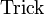$\mbox{Trick}{}_{}^{}$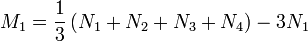$M_1=\frac{1}{3}\left(N_1+N_2+N_3+N_4\right)-3N_1$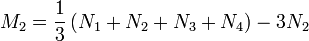$M_2=\frac{1}{3}\left(N_1+N_2+N_3+N_4\right)-3N_2$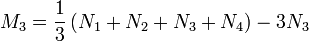$M_3=\frac{1}{3}\left(N_1+N_2+N_3+N_4\right)-3N_3$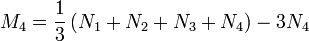$M_4=\frac{1}{3}\left(N_1+N_2+N_3+N_4\right)-3N_4$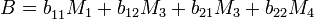$B=b_{11}^{}M_1+b_{12}M_3+b_{21}M_3+b_{22}M_4$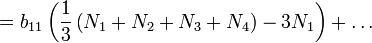$=b_{11}\left(\frac{1}{3}\left(N_1+N_2+N_3+N_4\right)-3N_1\right)+\ldots$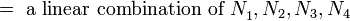$=\mbox{ a linear combination of }N_1^{},N_2,N_3,N_4$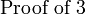$\mbox{Proof of 3}{}_{}^{}$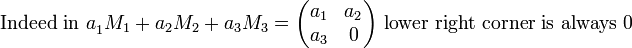$\mbox{Indeed in }a_1^{}M_1+a_2M_2+a_3M_3= \begin{pmatrix}a_1&a_2\\a_3&0\end{pmatrix}\mbox{ lower right corner is always } 0$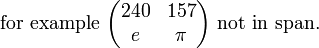$\mbox{for example }\begin{pmatrix}240&157\\e&\pi\end{pmatrix}\mbox{ not in span.}$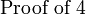$\mbox{Proof of 4}{}_{}^{}$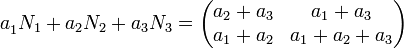$a_1^{}N_1+a_2N_2+a_3N_3=\begin{pmatrix}a_2+a_3&a_1+a_3\\a_1+a_2&a_1+a_2+a_3\end{pmatrix}$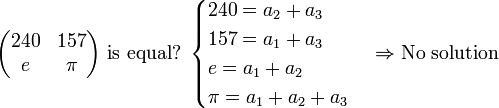$\begin{pmatrix}240&157\\e&\pi\end{pmatrix}\mbox{ is equal? } \begin{cases}240=a_2+a_3\\157=a_1+a_3\\e=a_1+a_2\\\pi=a_1+a_2+a_3\end{cases}\Rightarrow\mbox{No solution}$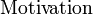$\mbox{Motivation}{}_{}^{}$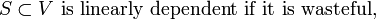$S\subset V\mbox{ is linearly dependent if it is wasteful,}$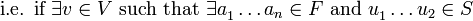$\mbox{i.e. if }\exists v\in V\mbox{ such that }\exists a_1^{}\ldots a_n\in F \mbox{ and }u_1^{}\ldots u_2\in S$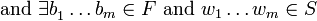$\mbox{ and }\exists b_1^{}\ldots b_m\in F \mbox{ and }w_1\ldots w_m\in S$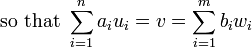$\mbox{so that }\sum_{i=1}^na_iu_i=v=\sum_{i=1}^mb_iw_i$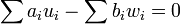$\sum a_iu_i-\sum b_iw_i=0$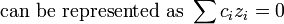$\mbox{can be represented as }\sum c_iz_i=0$$\mbox{Definition}{}_{}^{}$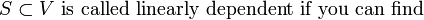$S\subset V\mbox{ is called linearly dependent if you can find }$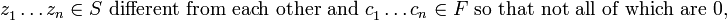$z_1^{}\ldots z_n\in S\mbox{ different from each other and }c_1^{}\ldots c_n\in F\mbox{ so that not all of which are } 0,$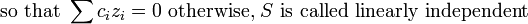$\mbox{so that }\sum c_iz_i=0 \mbox{ otherwise, }S\mbox{ is called linearly independent}$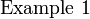$\mbox{Example 1}{}_{}^{}$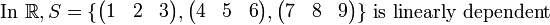$\mbox{In }\mathbb{R}, S= \lbrace\begin{pmatrix}1&2&3\end{pmatrix}, \begin{pmatrix}4&5&6\end{pmatrix}, \begin{pmatrix}7&8&9\end{pmatrix}\rbrace\mbox{ is linearly dependent}$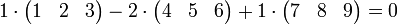$1\cdot\begin{pmatrix}1&2&3\end{pmatrix}- 2\cdot\begin{pmatrix}4&5&6\end{pmatrix}+ 1\cdot\begin{pmatrix}7&8&9\end{pmatrix}=0$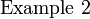$\mbox{Example 2}{}_{}^{}$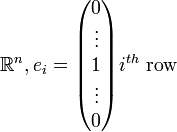$\mathbb{R}^n, e_i=\begin{pmatrix}0\\\vdots\\1\\\vdots\\0\end{pmatrix}i^{th}\mbox{ row}$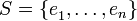$S=\lbrace e_1^{},\ldots,e_n\rbrace$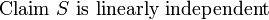$\mbox{Claim }S\mbox{ is linearly independent}{}_{}^{}$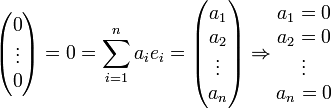$\begin{pmatrix}0\\\vdots\\0\end{pmatrix}=0 =\sum_{i=1}^na_ie_i =\begin{pmatrix}a_1\\a_2\\\vdots\\a_n\end{pmatrix}\Rightarrow \begin{matrix}a_1=0\\a_2=0\\\vdots\\a_n=0\end{matrix}$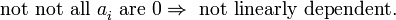$\mbox{not not all }a_i^{}\mbox{ are }0\Rightarrow \mbox{ not linearly dependent.}$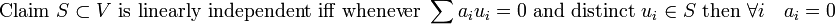$\mbox{Claim }S\subset V\mbox{ is linearly independent iff whenever }\sum a_iu_i=0 \mbox{ and distinct }u_i\in S\mbox{ then }\forall i\quad a_i=0$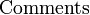$\mbox{Comments}{}_{}^{}$

1.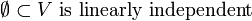$\emptyset\subset V\mbox{ is linearly independent}$
2.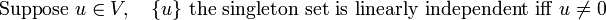$\mbox{Suppose }u\in V,\quad \lbrace u\rbrace\mbox{ the singleton set is linearly independent iff }u_{}^{}\neq 0$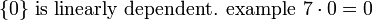$\lbrace0\rbrace\mbox{ is linearly dependent. example }7\cdot0=0$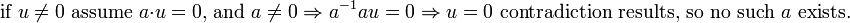$\mbox{if }u\neq0\mbox{ assume }a\cdot u=0\mbox{, and }a\neq0\Rightarrow a_{}^{-1}au=0 \Rightarrow u=0\mbox{ contradiction results, so no such }a\mbox{ exists.}$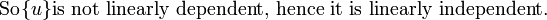$\mbox{ So}{}_{}^{}\lbrace u\rbrace\mbox{is not linearly dependent, hence it is linearly independent.}$